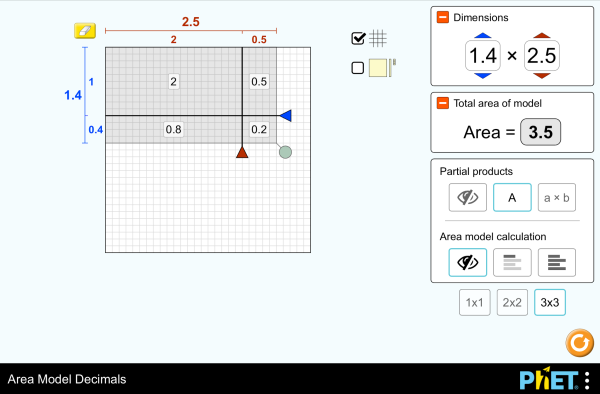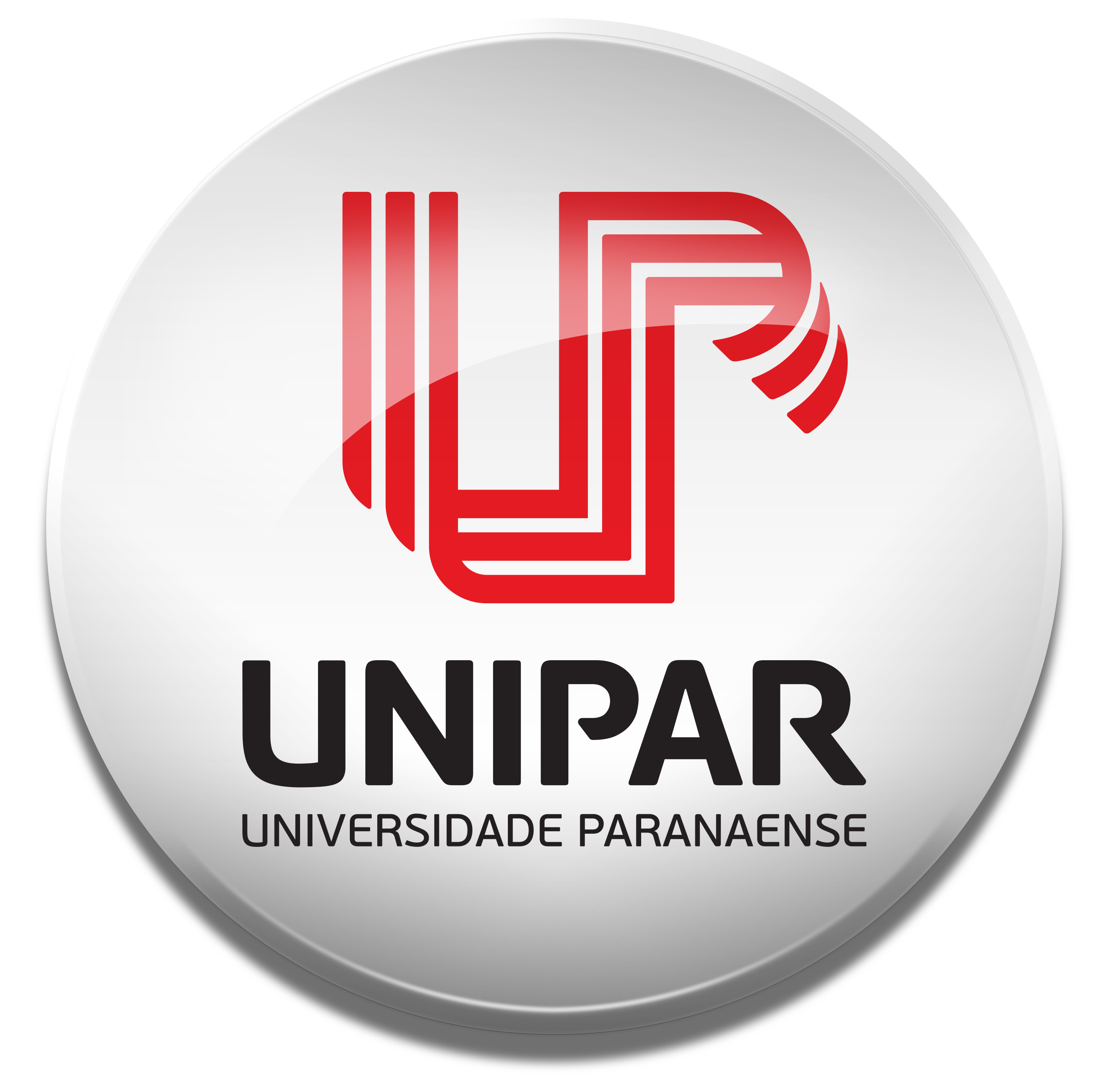# Területmodell: tizedesjegyekLetöltésBeágyazás bezárás A szimuláció futó példányának beágyazása Ezzel a HTML kóddal a szimuláció futó példányát ágyazhatja be weboldalába. A beágyazott szimuláció szélességét, ill. magasságát a "width", ill. a "height" paraméter értékének átállításával szabályozhatja. Olyan kép beágyazása a weboldalba, amelyre kattintva elindul a szimuláció
Kattintásra indul
Ezzel a HTML kóddal egy olyan képernyőfelvételt ágyazhat be weboldalába a szimulációról, melyen a "Kattintásra indul" szöveg olvasható. Szorzás Decimals Area Model A PhET egyik támogatójavalamint az Önhöz hasonló oktatók.

### Témák

• Szorzás
• Decimals
• Area Model
• Factors
• Partial Products

### Leírás

Build rectangles of various sizes and relate multiplication to area. Partition a rectangle into smaller areas and discover new strategies for multiplying decimals!

### Lehetséges tanulási célok

• Recognize that area represents the product of two numbers and is additive.
• Develop and justify a strategy that uses the area model to simplify a decimal multiplication problem.
• Devise a strategy to determine the product of two rational numbers (or an integer and a rational number) by representing the product as an area or the sum of areas.
• Use an area model to understand why multiplying a given number by a decimal less than 1 results in a product smaller than the given number.
• Interpret the product of two rational numbers using base-ten tiles

### Standards Alignment

#### Common Core - Math

5.NF.B.4
Apply and extend previous understandings of multiplication to multiply a fraction or whole number by a fraction.
5.NF.B.4a
Interpret the product (a/b) × q as a parts of a partition of q into b equal parts; equivalently, as the result of a sequence of operations a × q ÷ b. For example, use a visual fraction model to show (2/3) × 4 = 8/3, and create a story context for this equation. Do the same with (2/3) × (4/5) = 8/15. (In general, (a/b) × (c/d) = ac/bd.)
5.NF.B.4b
Find the area of a rectangle with fractional side lengths by tiling it with unit squares of the appropriate unit fraction side lengths, and show that the area is the same as would be found by multiplying the side lengths. Multiply fractional side lengths to find areas of rectangles, and represent fraction products as rectangular areas.
5.NF.B.5
Interpret multiplication as scaling (resizing), by:
5.NF.B.5a
Comparing the size of a product to the size of one factor on the basis of the size of the other factor, without performing the indicated multiplication.
5.NF.B.5b
Explaining why multiplying a given number by a fraction greater than 1 results in a product greater than the given number (recognizing multiplication by whole numbers greater than 1 as a familiar case); explaining why multiplying a given number by a fraction less than 1 results in a product smaller than the given number; and relating the principle of fraction equivalence a/b = (n × a)/(n × b) to the effect of multiplying a/b by 1.
Verzió 1.2.1

### Tanárok által megosztott aktivitások

Games Remote Lesson ideasTrish Loeblein Ó-5. o.
Kp.al.
Kp.em.
BSc.k.
Remote
Hf
Fizika
Kémia
Matematika
Area Model Decimals - Grade 6Maura Dudley Kp.al. Guided Matematika
Middle School Math Sim AlignmentAmanda McGarry Kp.al. Egyéb Matematika
PRIMARIA: Alineación con programas de la SEP México (2011 y 2017)Diana López Kp.al.
Ó-5. o.
Dem
Hf
Guided
Lab
Discuss
Matematika
Fizika
Kémia
Csillagászat
albán All shqipModeli i Syprinës: Decimal
angol All EnglishArea Model Decimals
arab All العربيةنموذج مساحة الأعداد العشرية
baszk All EuskaraAzalera-modeloa hamartarrekin
bosnyák All BosanskiMatematički model površina - Decimalni brojevi
dán All DanskArealmodel, decimaler
feröeri All FaroeseVíddir við desimalum
finn All suomiPinta-alamalli desimaaleilla
francia All françaisModélisation par les aires avec décimales
görög All ΕλληνικάΥπολογισμός εμβαδού - Δεκαδικοί
gujarati All Gujaratiક્ષેત્રફળનું મોડેલ - દશાંશ
hindi All हिंदीनमूने का क्षेत्रफल - दाशमिक
holland All NederlandsOppervlakte model decimalen
japán All 日本語面積と式の展開（小数）
kazah All KazakhАуданды ондық бөлшектермен есептеу
kínai (egyszerűsített) All 中文 (中国)面积模型：小数
kínai (hagyományos) All 中文 (台灣)Area Model Decimals_面積模型與小數
koreai All 한국어면적 모형: 소수
lengyel All polskiModel pola - ułamki dziesiętne
lett All LatviešuLaukuma modeļa decimāldaļskaitļi
marathi All मराठीअपूर्णांकातील क्षेत्रफळ
mongol All Монгол (Монгол)Талбайн Загвар Бутархайгаар
német All DeutschFlächenmodell Dezimalzahlen
olasz All italianoRappresentazione grafica del prodotto di numeri decimali
orosz All русскийВычисление площади с десятичными дробями
perzsa All فارسیمدل مساحت اعشاری ها
portugál (Brazília) All português (Brasil)Modelo de Área: Decimais
spanyol All españolModelo de Áreas: Decimales
spanyol (Mexikó) All español (México)Modelo de Áreas: Decimales
spanyol (Peru) All español (Perú)Modelo de área con decimales
svéd All svenskaAreamodellen och decimaltal
szerb All СрпскиМатематика преко површина-Децимални бројеви
szingaléz All Sinhaleseවර්ගඑල ආකෘති දශම
tamil All Tamilமாதிரிகளின் தசமப் பரப்பளவு
török All TürkçeAlan Modelinde Çarpma İşlemi (Ondalık)
ukrán All українськаПлощинна модель десяткових дробів
vietnami All Tiếng ViệtSố thập phân với mô hình diện tích

HTML5 sims can run on iPads and Chromebooks, as well as PC, Mac, and Linux systems.

iOS 12+ Safari

Android:
Not officially supported. If you are using the HTML5 sims on Android, we recommend using the latest version of Google Chrome.

Chromebook:
The HTML5 and Flash PhET sims are supported on all Chromebooks.
Chromebook compatible sims

Windows Systems:

Macintosh Systems:

Linux Systems:

Tervezőcsapat Külső könyvtárak Köszönet
• Jonathan Olson (developer)
• Karina Hensberry
• Susan Miller
• Ariel Paul
• Kathy Perkins
• almond-0.2.9.js
• base64-js-1.2.0.js
• FileSaver-b8054a2.js
• font-awesome-4.5.0
• game-up-camera-1.0.0.js
• he-1.1.1.js
• himalaya-0.2.7.js
• jama-1.0.2
• jquery-2.1.0.js
• lodash-4.17.4.js
• pegjs-0.7.0.js
• seedrandom-2.4.2.js
• text-2.0.12.js
• TextEncoderLite-3c9f6f0.js